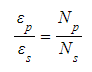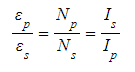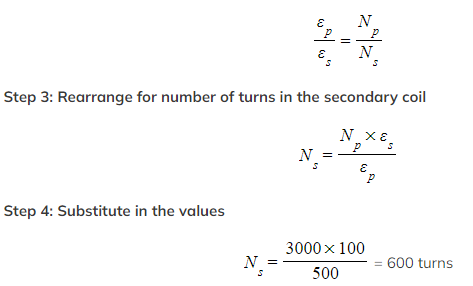# IB DP Physics: HL复习笔记11.2.4 Transformer Calculations

### Transformer Calculations

• The transformer equation is:
•Where:
• Ns = number of turns in the secondary coil
• Np = number of turns in the primary coil
• εs = output voltage from the secondary coil (V)
• εp = input voltage in the primary coil (V)

There are two types of transformers:

• Step-up transformer (increases the voltage of the power source) where Ns > Np
• Step-down transformer (decreases the voltage of the power source) where Np > Ns
• For an ideal transformer, there is no electrical energy lost and it is 100% efficient
• This means the power in the primary coil equals the power in the second coil;• Where:
• Ip = current in the primary coil (A)
• Is = output current from the secondary coil (A)

#### Worked Example

A step-down transformer turns a primary voltage of 0.5 kV into a secondary voltage of 100 V. Calculate the number of turns needed in the secondary coil if the primary coil contains 3000 turns of wire.

Step 1: List the known quantities

• Primary voltage, εp = 0.5 kV = 0.5 × 103 V
• Secondary voltage, εs = 100 V
• Number of turns in the primary coil, Np = 3000 turns

Step 2: Write down the transformer equation